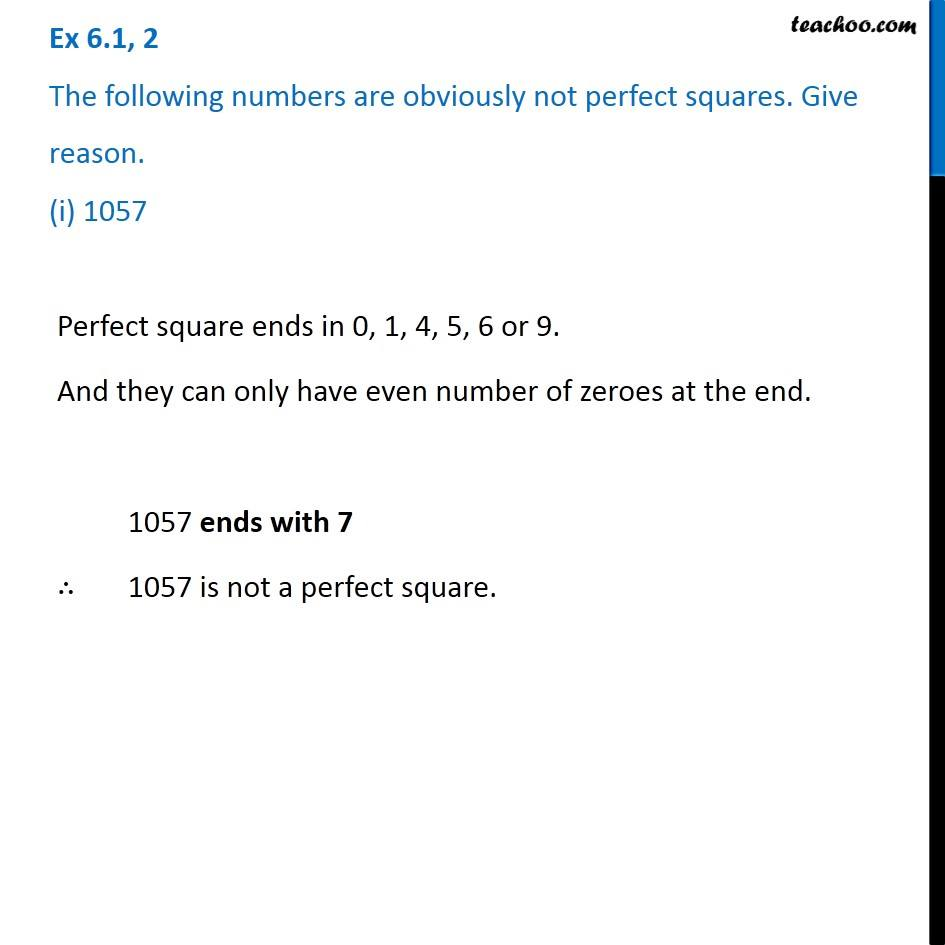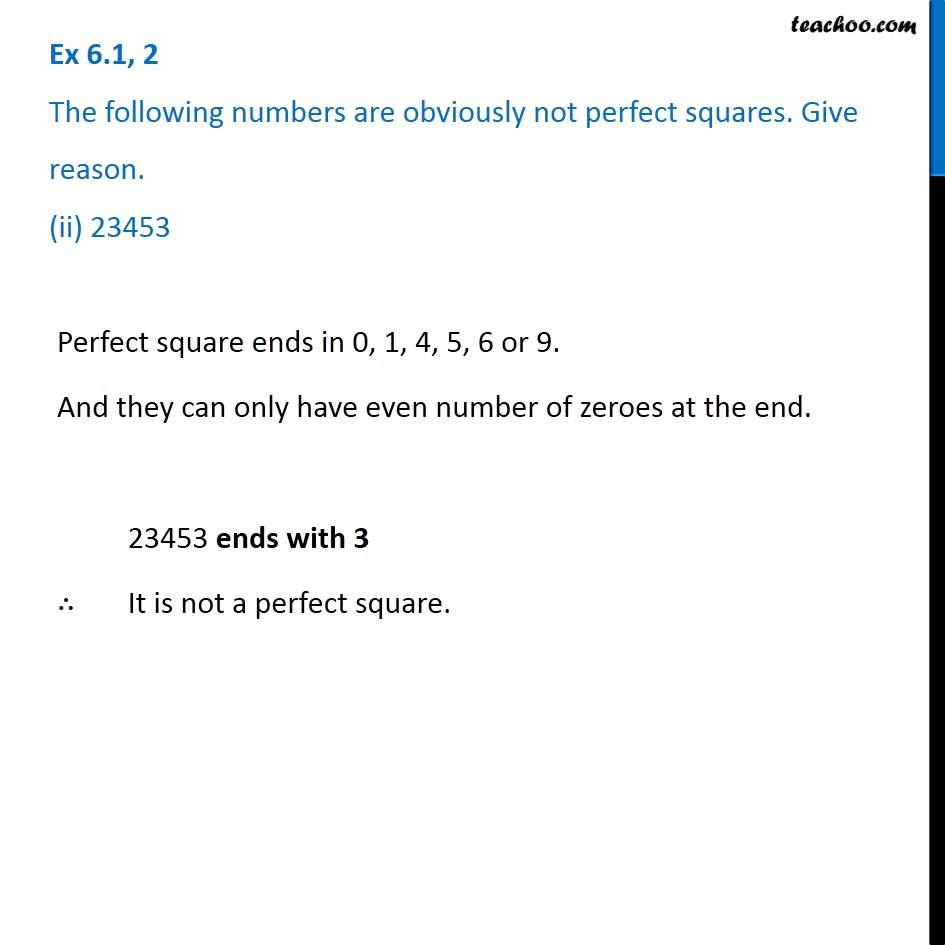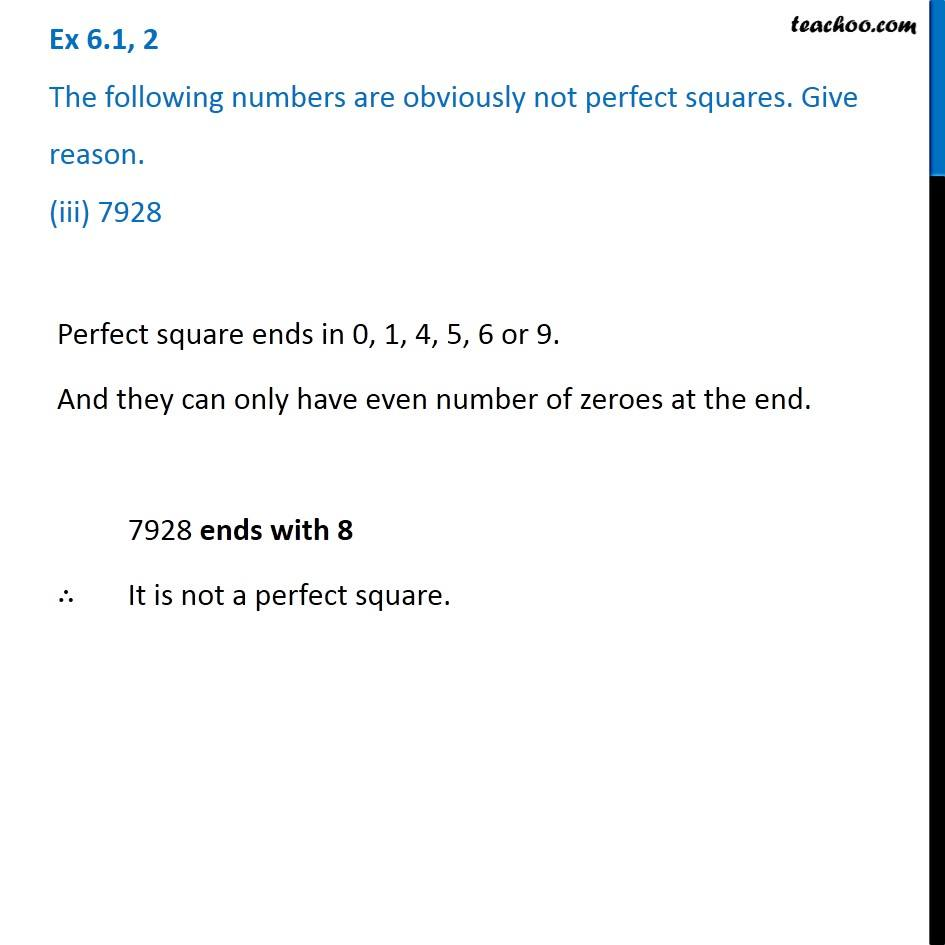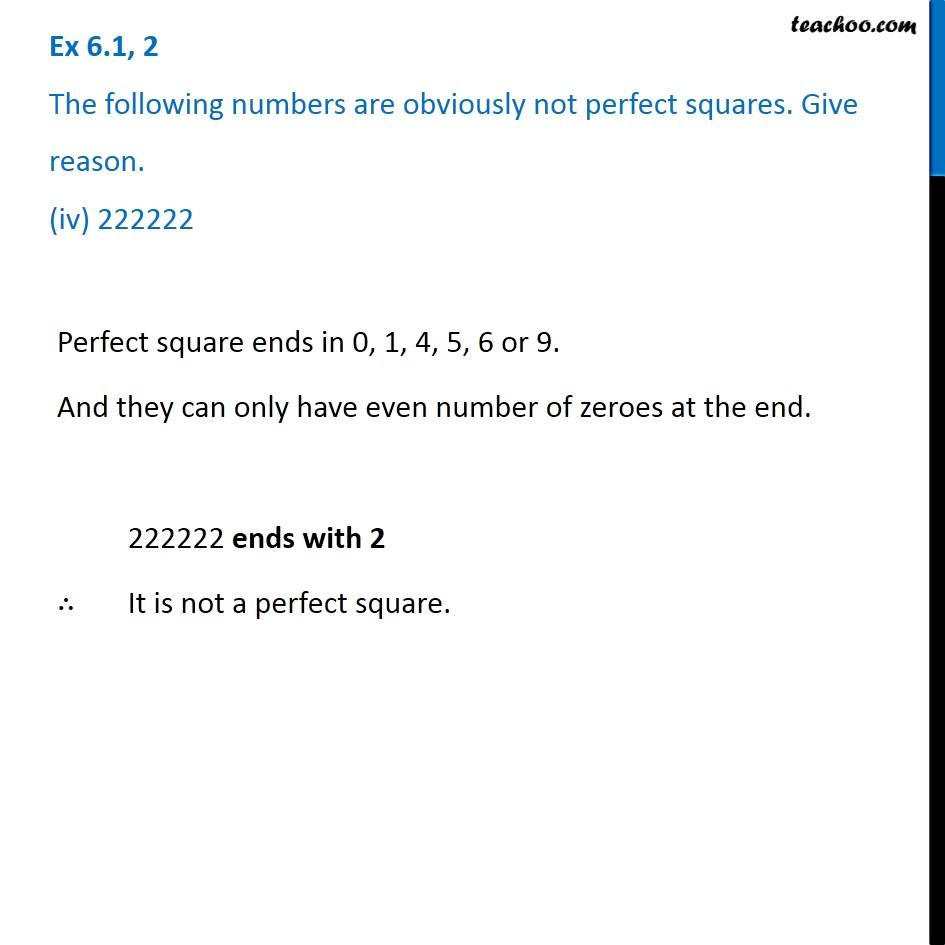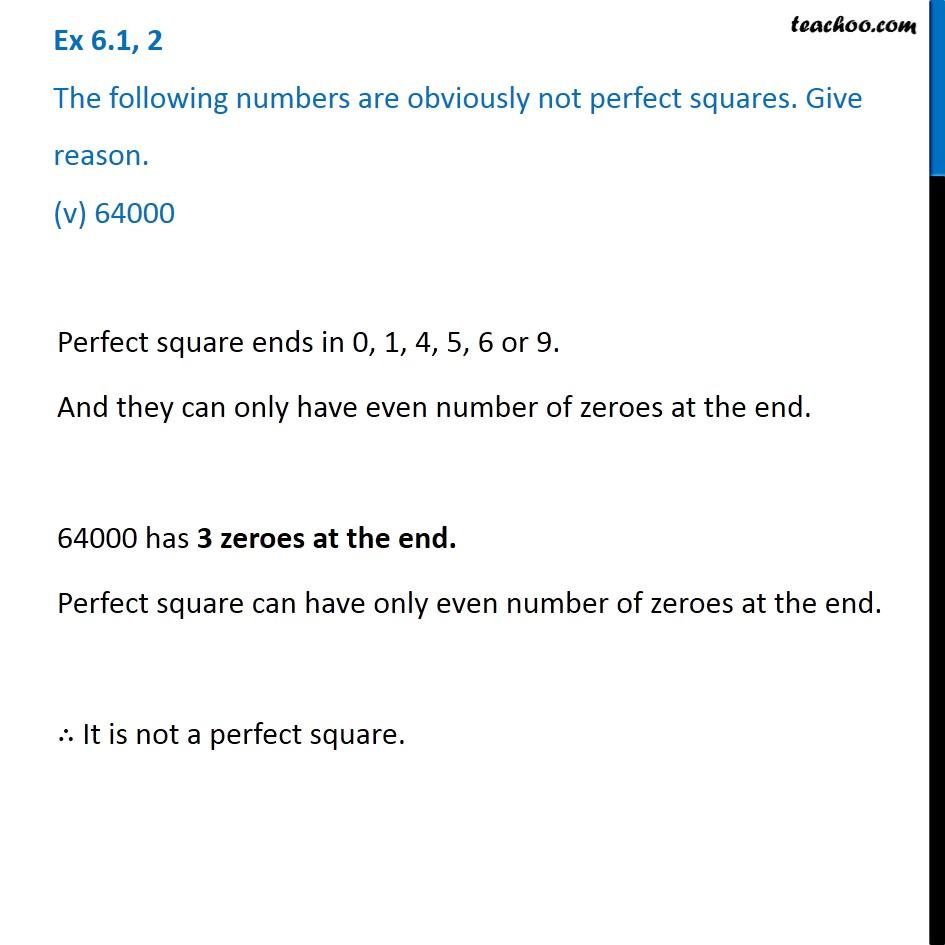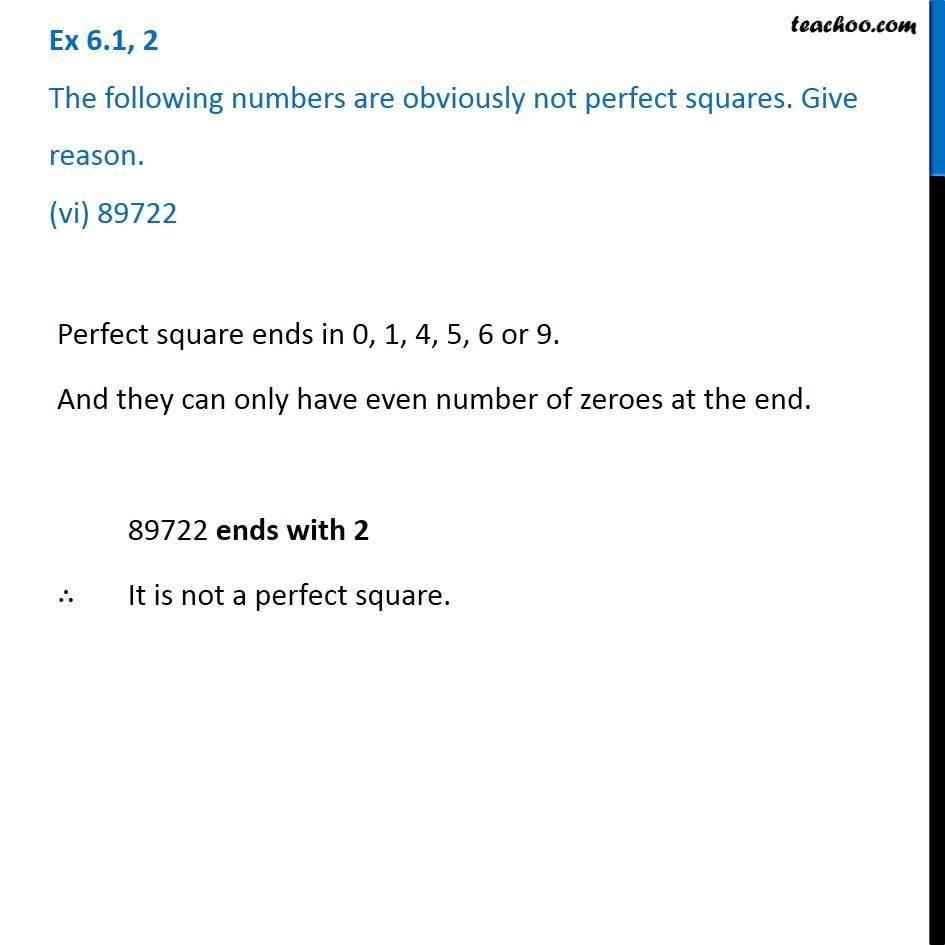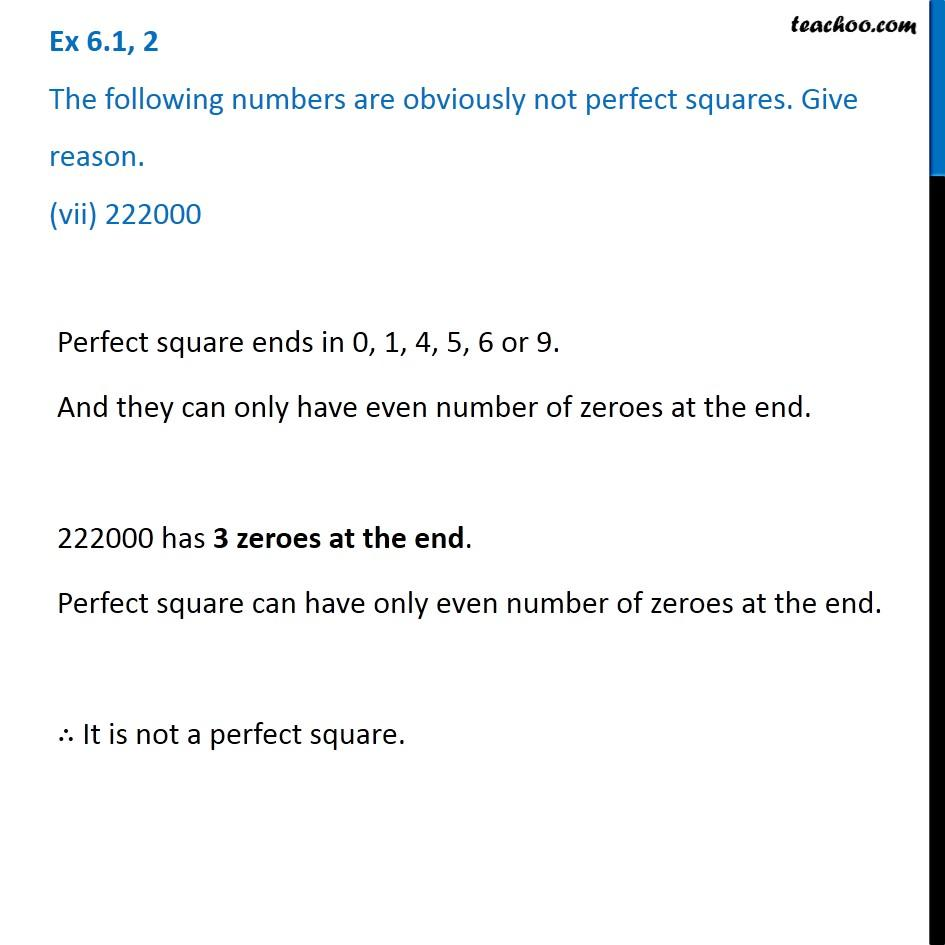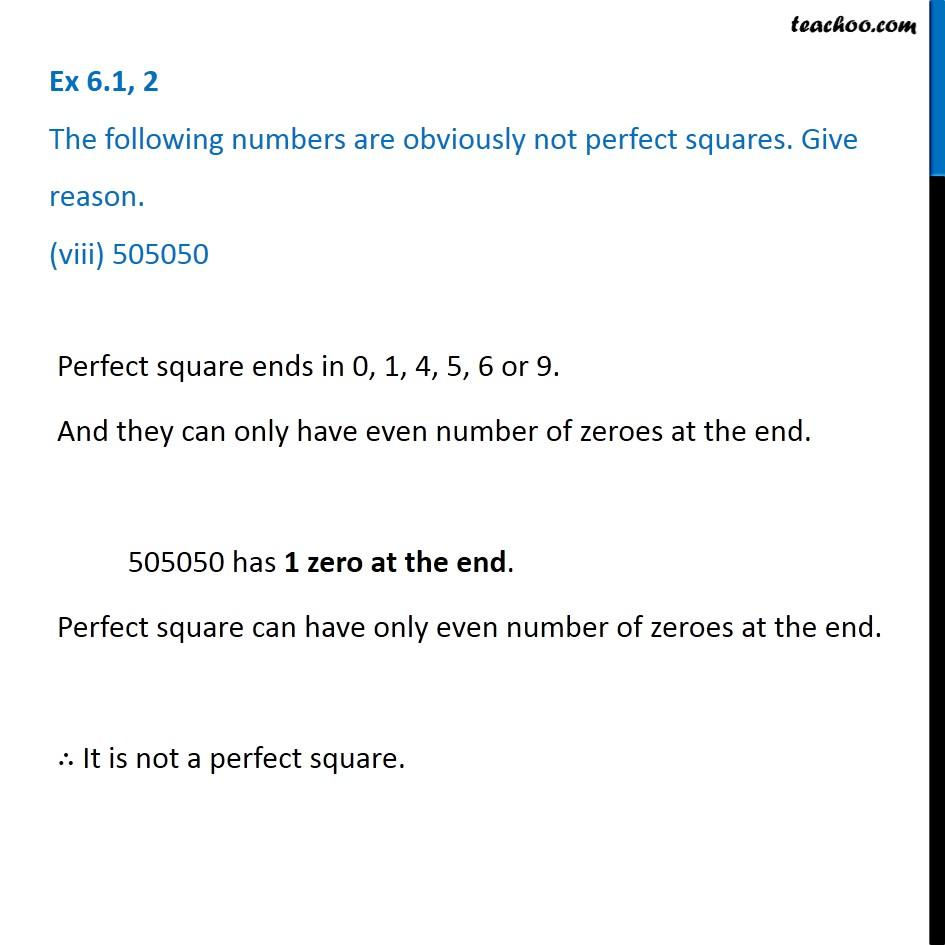Subscribe to our Youtube Channel - https://you.tube/teachoo

1. Chapter 6 Class 8 Squares and Square Roots
2. Serial order wise
3. Ex 6.1

Transcript

Ex 6.1, 2 The following numbers are obviously not perfect squares. Give reason. (i) 1057 Perfect square ends in 0, 1, 4, 5, 6 or 9. And they can only have even number of zeroes at the end. 1057 ends with 7 ∴ 1057 is not a perfect square. Ex 6.1, 2 The following numbers are obviously not perfect squares. Give reason. (ii) 23453 Perfect square ends in 0, 1, 4, 5, 6 or 9. And they can only have even number of zeroes at the end. 23453 ends with 3 ∴ It is not a perfect square. Ex 6.1, 2 The following numbers are obviously not perfect squares. Give reason. (iii) 7928 Perfect square ends in 0, 1, 4, 5, 6 or 9. And they can only have even number of zeroes at the end. 7928 ends with 8 ∴ It is not a perfect square. Ex 6.1, 2 The following numbers are obviously not perfect squares. Give reason. (iv) 222222 Perfect square ends in 0, 1, 4, 5, 6 or 9. And they can only have even number of zeroes at the end. 222222 ends with 2 ∴ It is not a perfect square. Ex 6.1, 2 The following numbers are obviously not perfect squares. Give reason. (v) 64000 Perfect square ends in 0, 1, 4, 5, 6 or 9. And they can only have even number of zeroes at the end. 64000 has 3 zeroes at the end. Perfect square can have only even number of zeroes at the end. ∴ It is not a perfect square. Ex 6.1, 2 The following numbers are obviously not perfect squares. Give reason. (vi) 89722 Perfect square ends in 0, 1, 4, 5, 6 or 9. And they can only have even number of zeroes at the end. 89722 ends with 2 ∴ It is not a perfect square. Ex 6.1, 2 The following numbers are obviously not perfect squares. Give reason. (vii) 222000 Perfect square ends in 0, 1, 4, 5, 6 or 9. And they can only have even number of zeroes at the end. 222000 has 3 zeroes at the end. Perfect square can have only even number of zeroes at the end. ∴ It is not a perfect square. Ex 6.1, 2 The following numbers are obviously not perfect squares. Give reason. (viii) 505050 Perfect square ends in 0, 1, 4, 5, 6 or 9. And they can only have even number of zeroes at the end. 505050 has 1 zero at the end. Perfect square can have only even number of zeroes at the end. ∴ It is not a perfect square.

Ex 6.1

About the AuthorDavneet Singh
Davneet Singh is a graduate from Indian Institute of Technology, Kanpur. He has been teaching from the past 9 years. He provides courses for Maths and Science at Teachoo.